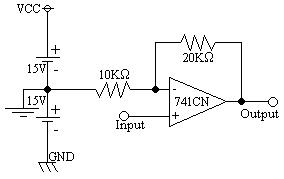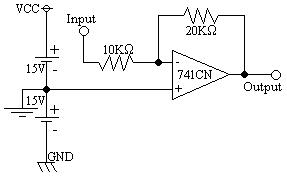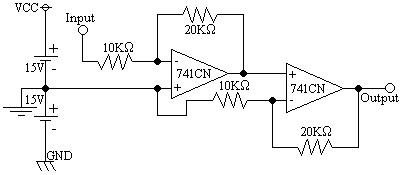EL - 250

Lab #4: The Operational Amplifier

Andrew Buettner

March 5, 2003Objective

The objective of this lab is to construct several amplifier circuits using operational amplifiers, then to test the operation of those amplifiers and compare the results with theoretical values obtained through calculation.Equipment Used

1) Trainer #

2) Multimeter #

3) Oscilloscope #

4) Audio function generator #

5) Various components from the EL - 250 lab kitProcedure

A: Inverting Op - Amp Amplifier

1) Assemble the following circuit:2) Set the input voltage to 2VAC.

3) Measure the voltage produced at the output.

4) Calculate the theoretical voltage at the output and compare the two results.

B: Non - inverting Op - Amp Amplifier

1) Assemble the following circuit:2) Set the input voltage to 2VAC.

3) Measure the voltage produced at the output.

4) Calculate the theoretical voltage at the output and compare the two results.

C: Multiple Op - Amp Amplifier

1) Assemble the following circuit:2) Set the input voltage to 2VAC.

3) Measure the voltage produced at the output.

4) Calculate the theoretical voltage at the output and compare the two results.Data

1) Diagram 1: Schematic 1 Enlarged View2) Diagram 2: Schematic 2 Enlarged View3) Diagram 3: Schematic 3 Enlarged View4) Table 1: Voltage Results (`-' means 180o out of phase)

Amplifier type:

Actual

Theoretical

% Error

Inverting

-4V

-4V

0.00%

Non-inverting

6V

6V

0.00%

-17V

-24V

29.2%Conclusions

From this lab it is concluded that an Operational Amplifier can be used to create a working amplifier that exhibits a predicable pattern. In addition to the tests that I performed in accordance with the lab, I also tested the amplifier with other functions (square wave, triangle wave, sawtooth wave), and it performed as exceptionally. I am pleased to find that the operational amplifier follows the mathematical equations very well. Although I am not sure of the error in the cascaded amplifier, there may have just been an errant observation.Attachments

1. Original lab handout

2. Original lab data

3. Calculations